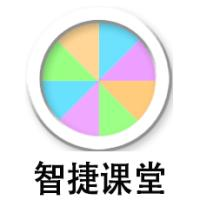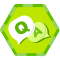## Swift使用闭包表达式 原

Swift中的闭包表达式很灵活，其标准语法格式如下：
{ (参数列表) ->返回值类型 in
语句组
}

Swift提供了多种闭包简化写法，这一节我们将介绍几种不同的形式。
1.类型推断简化

{(a:Int, b:Int) -> Int in
return a + b
}
Swift能推断出参数a和b是Int类型，返回值也是Int类型。简化形式如下：
{a, b in return a + b }

[html] view plaincopy

1. func calculate(opr :String)-> (Int,Int)-> Int {

2.

3.

4.     var result : (Int,Int)-> Int

5.

6.     switch (opr) {

7.     case "+" :

8.         result = {a, b in return a + b }　　　　　　　　①

9.     default:

10.         result = {a, b in return a - b }　　　　　　　　②

11.     }

12.     return result;

13. }

14.

15.

16. let f1:(Int,Int)-> Int = calculate("+")

17. println("10 + 5 = \(f1(10,5))")

18.

19.

20. let f2:(Int,Int)-> Int = calculate("-")

21. println("10 + 5 = \(f2(10,5))")

2.隐藏return关键字

{a, b in a + b }

[html] view plaincopy

1. func calculate(opr :String)-> (Int,Int)-> Int {

2.

3.

4.     var result : (Int,Int)-> Int

5.

6.     switch (opr) {

7.     case "+" :

8.         result = {a, b in return a + b }　　　　　　　　①

9.     default:

10.         result = {a, b in return a - b }　　　　　　　　②

11.     }

12.     return result;

13. }

{a, b in var c; a + b }
3.缩写参数名称

{\$0 + \$1}

[html] view plaincopy

1. func calculate(opr :String)-> (Int,Int)-> Int {

2.

3.

4.     var result : (Int,Int)-> Int

5.

6.     switch (opr) {

7.     case "+" :

8.         result = {\$0 + \$1}  ①

9.     default:

10.         result = {\$0 - \$1}  ②

11.     }

12.     return result;

13. }

14.

15.

16. let f1:(Int,Int)-> Int = calculate("+")

17. println("10 + 5 = \(f1(10,5))")

18.

19.

20. let f2:(Int,Int)-> Int = calculate("-")

21. println("10 + 5 = \(f2(10,5))")

4.使用闭包返回值

[html] view plaincopy

1. let c1:Int = {(a:Int, b:Int) -> Int in

2.                 return a + b

3.           }(10,5)       ①

4.

5.

6. println("10 + 5 = \(c1)")

7.

8.

9. let c2:Int = {(a:Int, b:Int) -> Int in

10.                     return a - b

11.                 }(10,5)     ②

12.

13.

14. println("10 - 5 = \(c2)")### 智捷课堂《从零开始学Swift》学习笔记（Day 22）——闭包那些事儿！

2015/10/14
33
0
Swift讲解专题八——闭包

Swift讲解专题八——闭包 一、引言 Swift中的闭包是有一定功能的代码块，这十分类似于Objective-C中的block语法。Swift中的闭包语法风格十分简洁，其作用和函数的作用相似。 二、从一个系统函...

2016/05/14
885
3
Swift2.0语言教程之闭包

Swift2.0语言教程之闭包 Swift2.0语言闭包 闭包是自包含的函数代码块，可以在代码中被传递和使用。Swift中的闭包与C和Objective-C中的代码块（blocks）以及其他一些编程语言中的Lambda函数比...

2015/07/20
207
0
RxSwift之路 1#Swift语法知识准备

2017/07/11
0
0
Swift3.0 闭包(blcok)的全面介绍及使用

2016/12/28
99
0

c++ 内联函数调用快的原因

42分钟前
4
0
JS--function

wytao1995

5
0

7
0

zzxworld

6
0
sonar报错volatile

5
0